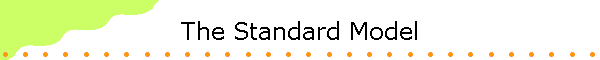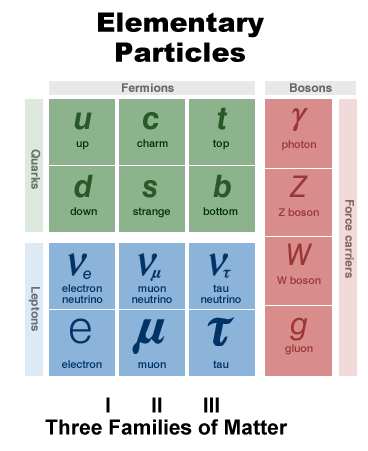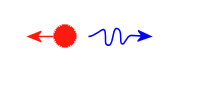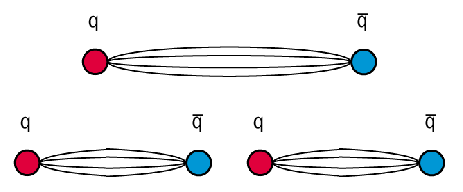Quantum Mechanics is our current model to describe how the microscopic world works.  We have studied the implications of the Schroedinger equation, which is a fundamental equation for the non-relativistic version of quantum mechanics.  Particles are neither created nor destroyed in this version.  To apply quantum mechanics to particle physics, the relativistic version of quantum mechanics, called quantum field theory (QFT), must be used.  QFT is well suited to let us calculate probabilities of creation and annihilation of particles in interactions.  QFT is used to study scattering, decay, and vacuum fluctuations, but seldom to study bound states, which quantum mechanics handles well.

To use quantum field theory to predict the behavior of fundamental particles, we need to understand the interactions (or forces) between the particles.  We need a mathematical model for those interactions.  The known forces are the electromagnetic, the strong, the weak, and the gravitational force.  Fundamental or elementary particles are those that, as far as current experiments can tell, have no substructure.  These are the quarks and leptons, and the force carriers for the known forces.Force carriers

In quantum field theory, forces are transmitted by particles, and fields are associated with particles which transmit the forces.  The particles of the electromagnetic field are the photons.  In quantum electrodynamics all electromagnetic fields are associated with photons, and the interaction between the charged particles occurs when one charged particle emits a virtual photon that is then absorbed by another charged particle.  The photon has to be a virtual photon, because emission of a real photon would violate energy and momentum conservation.  If, for example, an electron initially at rest emitted a photon, the final state would consist of an electron and a photon moving off in opposite directions, a configuration which necessarily has more energy than the initial at-rest electron.Particle E = mc2 Particle + Photon E = mc2 + kinetic energy + hf

But the uncertainty principle prevents a contradiction.  The uncertainty relation, ΔEΔt ~ ћ, tells us that if we only observe a system for a time interval Δt, there is no way that we can know the energy of the system better than to within an uncertainty ΔE.  So the photon can have energy ΔE for a time interval Δt ~ ћ/ΔE, without anybody being able to know if energy conservation is violated.  As long as the photon is reabsorbed quickly enough, there is no measurable violation of energy conservation.  Since the photon must be reabsorbed and cannot be detected, it is called a virtual photon.  A QFT calculation of the effect of this virtual photon exchange gives rise to the usual Coulomb force.  Like charges repel and unlike charges attract.

A virtual photon can exist for a time Δt ~ ћ/ΔE.  Since for a photon the wavelength can be arbitrarily large and therefore the energy arbitrarily small, we can have ΔE --> 0,  Δt --> infinity.  The virtual photon can have an infinite range.

To explain the attractive nuclear force between nucleons, Yukawa proposed the existence of a meson field, whose quanta would be emitted and absorbed by nucleons and therefore transmit the strong interaction.  The fact that the nuclear force is a short-range force leads to an important difference between photons and the new quanta.  The new quanta must be massive particles.

Δt ~ ћ/ΔE  ~ћ/mc2

ΔE ~ mc2 since the particle either exists or does not exist.  The virtual particle can propagate a distance no larger than R = cΔt in a time interval Δt.  If we insert Δt ~ ћ/ΔE from above, we have  R ~ ћ/mc, or m ~ ћ/Rc.  From the known range of the strong force, we can now estimate the mass of the particles that transmits it.  Therefore Yukawa suggested that a meson with a mass intermediate between that of an electron and a proton is exchanged between nucleons.  The subsequent discovery of pions had a lasting influence on how we now think about the fundamental interactions, even if we no longer think of the exchange of pions as representing a fundamental interaction.  We now think of all fundamental interactions as being transmitted by particles, which we call the force carriers.  At a fundamental level, a force isn't just something that happens to particles.  It is a thing which is passed between two particles

Particles that can interact via a certain kind of interaction continuously emit and absorb virtual particles, the force carriers for that interaction.  They are surrounded by a cloud of these virtual particles.  A particular force carrier particle can only be absorbed or produced by a particle which is affected by that particular force.  For instance, electrons and protons have electric charge, so they can produce and absorb the electromagnetic force carrier, the photon.  Neutrinos, on the other hand, have no electric charge, so they cannot absorb or produce photons.

Charged particles interact via electromagnetic fields.  Charged particle produce electric fields and are acted on by electric fields.  Moving charged particles also produce magnetic fields and are acted on by magnetic fields.  Accelerating charged particles produce electromagnetic radiation.  In classical physics, the electromagnetic interactions are well understood.  The classical theory was developed by James Clerk Maxwell between 1861 and 1865 and is described by a set of equations called Maxwell's equations.  The electromagnetic fields explain how charged particle can interact over large distances.

Quantum electrodynamics (QED) is the QFT of the electromagnetic interaction.  It is the quantum version of classical electromagnetism.  Quantum electrodynamics describes precisely how charged particles interact through the exchange of photons.  By itself, this description is the most accurate theory that we know of.  For example, it predicts the magnetic moments of electrons and muons to an accuracy of about one part in a billion.

In QED charged particles can emit and absorb virtual photons.  Although these virtual photons cannot be observed directly, they contribute measurably to the probabilities of observable events.  But some calculations can lead to infinities.  To avoid mathematical inconsistencies and correct unphysical results, the technique of renormalization is used.  For example, the effective mass of electrons is modified by including the energy contributions of virtual photons.  Renormalization allows for setting the effective mass equal to the observed mass without violating known principles of physics.  In general, renormalization can remove infinities from the theory by absorbing the infinities into available free parameters without violating known principles of physics.

The Electroweak Force

Electromagnetism is the result of the unification of two forces that were initially thought to be independent, namely electric and magnetic forces.  The electroweak force is the result of the unification of electromagnetism and the weak force.  The weak interaction is responsible for beta decay.  Beta decay produces a neutrino, which does not interact via the strong or electromagnetic force.  So even though the weak force is extremely weak, it produces reactions that otherwise could not occur at all.  The weak force has an extremely short range R ~ 10-18 m.  Massive particles (m ~ ћ/Rc ~ 100 mproton ) therefore must transmit the weak force.

In the late 1960s it became clear that the weak interactions were on some level very similar to the electromagnetic interactions.  There is, of course, a difference in strength and there is a huge difference in range.  These differences were reconciled by S. Weinberg, A. Salam, and S. Glashow, who recognized that there was a single more fundamental interaction, the electroweak interaction.  They proposed that at high energies, above some critical energy, the weak interactions should be almost identical to the electromagnetic interactions, with a triplet of massless spin-1 particles acting as carriers of the force.  Two of these particles are charged and one is neutral.  These particles are the weak bosons, W+, W-, and Z0.   At first, the quantum field theory of electroweak interactions did not seem to have much going for it.  There was no model for how the weak bosons acquired their mass.  Adding the masses "by hand" made the theory not renormalizable.  The Z0 also had not been observed.

In 1971 Martinus Veltman and Gerardus 't Hooft succeeded to make the QFT of the electroweak interactions renormalizable.  They had to introduce at least one new interaction and a corresponding new particle to cancel out infinities in the theory.  The simplest interaction which makes the theory renormalizable is the Higgs field, which pervades the vacuum and fills the universe.  Interactions between intrinsically massless particles and the Higgs field make the particles acquire an observable mass.  The particle of the Higgs field is the neutral spin-0 Higgs boson, which is the last particle that must be added to the elementary particle table of the Standard Model.  The Higgs particle has not been detected yet and its mass is not known

In the QFT of the electroweak interactions the weak bosons W+, W-, and Z0 acquire large masses, approximately 86 and 97 times the mass of a proton for the Ws and Z0, respectively at energies below a critical value, that is, the particles behave as though they had mass due to their own interactions with the Higgs field.  The weak bosons feel a "drag" causing them to slow down from the speed of light.  In order to travel slower than the speed of light they must have mass.  The large mass of the weak bosons explains the very short range of the weak interaction.  The large mass also has the effect of making low energy processes in which exchange of weak bosons is involved occur at a much slower rate, thus explaining the "weakness" of the force.  The unification manifests itself only at very high energies, beyond our current ability to test directly, but even at the highest energies achievable now we can observe effects of the unification coming into play, thus giving experimental confirmation that these ideas are correct.

Processes involving the weak force can be thought of in terms of exchange of the weak bosons, subject to the conservation of electric charge, of baryon number, and lepton number.  Thus the underlying basis of beta decay, namely

d --> u + e- + νe.

can be visualized as a two-step process in which a d-quark within a neutron is transformed to a u-quark with emission of a W-, while the W- then transforms into two leptons.

d --> u + W-,     W- --> e- + νe.

The weak interaction is the only interaction that can change the flavor of a quark.   Quark flavor never changes except through weak interactions, like beta decay, that involve W bosons.  A quark type can convert to anotherr quark type with a different electric charge by emitting or absorbing a W boson.

Many questions remain.  Is there only one Higgs particle?  It is possible to renormalize the theory with more than one additional interaction.  If only one interaction is used, then the ratio of the mass of the Ws to the Z0 must have a well-defined value equal to the observed value.  Most likely there is only one Higgs boson.  What is its mass?   There are some predictions.  The measured ratio of the mass of the Ws to the Z0 should be slightly affected by the interactions of these particles with the top and bottom quarks, which depend on the masses of those quarks.  This rather small effect was used to predict the mass of the top quark before its discovery in 1995.  Once the mass of the top quark had been measured, the correction to the W/Z0 mass ration could be calculated exactly.  A much smaller discrepancy remained.  If this discrepancy is attributed to the difference in the strength of the interaction of these particles with the Higgs boson, which depends on the mass of the Higgs, a not very precise prediction of the Higgs mass can be made.  This leads to a prediction of somewhere above 110 GeV, with a large uncertainty.

The Strong Force

Our ideas about the strong force are based on the theory of quantum chromodynamics (QCD), a quantum field theory that describes interactions between quarks and a set of particles called gluons, with spin 1, that act as the carrier particles.  Quarks interact via electromagnetic, weak, and strong interactions.  They carry electrical charge, but all quarks, whether u, d, s, c, b, or t carry an additional, non-electrical charge, called the color charge.  Similar to the electric charge being responsible for the electromagnetic interaction, color charge is responsible for the strong interaction.  It is analogous to the electric charge in that, like electric charge, it is both quantized and conserved.  The label for the color charge is a bit more complicated than + and -, because the color charge occurs in three different types, red, green, and blue.  These labels have nothing to do with the colors of the visible-light spectrum, they merely  help us keep track of the charge.  In addition, for each color charge there exists an anti-color charge.

 Color Charge r g b r g b

Each flavor of a quark can have three possible color charges  But any baryon, which always is a three-quark state, contains one quark of each color and is a color-neutral.  This is the reason for the label "color charge".  Mixing three primary colors of paint to give white (or colorless paint) is an analogy for the color neutral combination of three quarks.  Any state of three anti-quarks (any anti-baryon) is also color neutral.  A quark of one of the three colors and an anti-quark of the corresponding anti-color make mesons color neutral.  All observed particles are color neutral.

Gluons are emitted and absorbed by particles with color charge, just as photons are emitted and absorbed by particles with electric charge.  They transmit the color force.  Gluons carry one color and one anti-color.  When a quark emits a gluon, it can change color.  If a red quark changes color and becomes a green quark, it emits a gluon that carries the colors red and anti-green.  The process of gluon emission and absorption occurs only inside hadrons and is not directly observable.

There is a crucial difference between QED and QCD.  Photons do not carry electric charge, but gluons carry the color quantum charge and can themselves interact via the strong force through the exchange of other gluons.  Gluons attract each other.  This difference is crucial, and makes the forces between quarks and gluons different from other forces we have studied before.  One effect of this interaction is that single quarks cannot be isolated.  When the interaction between a quark and an anti-quark is worked out, it turns out that the gluon field lines, analogous to the electromagnetic field lines in the interaction between opposite charges, do not spread as for an electric dipole but rather stay in tube-like regions, connecting quarks with anti-quarks.  Instead of being spread out all over space, the field energy is confined to a roughly cylindrical volume.  Separating the quark from the anti-quark requires more and more energy as the separation increases.  When the quark and anti-quark are pulled apart, the cylinder breaks creating a new quark - anti-quark pair.  Thus if one tries to separate a π-meson into its constituent quarks, one instead ends up breaking it up into two mesons.Even though the theory of quantum chromodynamics is well developed, we do not know how to calculate all the processes that are governed by it, largely because of a lack of mathematical tools.  The technical details simplify at high energies.  Calculating QCD contributions to high-energy scattering processes, when quarks get very close to each other, have become routine, since at short distances  the color force becomes weak, and perturbative approximation methods can be used.  The behavior of the color force at high energy or short distances is called asymptotic freedom.  At low energies some processes are easier to calculate than others.  In spite of these difficulties, there seems to be little doubt that quantum chromodynamics is the correct theory of the strong interactions.

The Standard Model

Particle physicists now believe they can describe the behavior of all known subatomic particles within a single theoretical framework called the Standard Model.  This model incorporates the quarks and leptons as well as their interactions through the strong, weak and electromagnetic forces.  The standard model puts the field theories QED and QCD under one umbrella.  Gravity remains outside the Standard Model.

According to the Standard Model, the basic forces are transmitted between the quarks and leptons by a third family of particles.  These are called gauge bosons, and they differ fundamentally from the quarks and leptons, which are the building blocks of matter.  There is a different type of particle for each force.  Photons carry the electromagnetic force, gluons carry the strong force, weak bosons carry the weak force.  A particle called the graviton is believed to be responsible for gravity, but it has not yet proved possible to build a self-consistent theory that contains the graviton.  The fundamental forces appear to behave very differently in ordinary matter, but the Standard Model indicates that they are basically very similar when matter is in a high-energy environment.

To the extent that we can perform the calculations necessary to make a prediction and perform the experiments necessary to test those predictions, the standard model has passed every test.  The standard model contains many parameters whose origins and values one would like to understand more deeply.  In this way, the standard model sets out a firm platform from which searches for "new physics" can be launched.  The standard model is not really a single theory of both electroweak and strong forces as much as it is a way to bring these two theories together under a single umbrella.  One important aspect of the standard model is that the theories of electroweak and strong forces are constructed in identical ways.  There are of course differences.  These differences lead to massive carriers for the weak interactions, massless carriers for the electromagnetic and strong interactions, and to confined gluons and quarks.  Yet in many ways there are more similarities than differences, and these have led to attempts to construct a single unified theory.

The Standard Model still contains many arbitrary parameters that come from experiments.  In the Standard Model, the quarks, leptons and gauge bosons acquire their masses through a mechanism devised by Peter Higgs of Edinburgh University.  According to this mechanism, particles interact with a new particle, known as the Higgs boson, and it is the strength of this interaction that gives the particles their masses.  The gauge bosons, which transmit the fundamental forces, are a manifestation of the fields associated with those forces.  In the same way, the Higgs boson is also the particle of a field.  However, this field has different properties from the others, in particular it gives rise to mass.  As yet we have no experimental evidence for the Higgs boson.  This is a missing link in the Standard Model, which otherwise stands up to very precise and stringent tests.  A major task for particle physics in the years to come will be to search for the missing ingredient, be it the Higgs boson or maybe something else, that underlies the origin of mass.Click on the picture to download a print-quality Fundamental Particles and Interactions Chart.